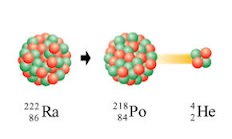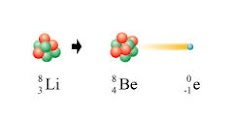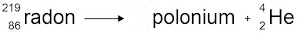Which of these is not a natural source of background radiation? *
1 point
A teacher used a Geiger counter to measure the radiation emitted by a radioactive rock. Wrapping the rock in paper and aluminium foil had no effect on the count rate. Which statement is true? *
1 point
The half-life of an isotope is 1 hour. How long will it take the count rate to fall to one quarter of the starting rate? *
1 point
What happens to the half life of a substance when it is put into a freezer? *
1 point
Which combination of features would be best when choosing a radioactive source for use a medical tracer?
1 point
What type of radioactive decay is shown below?
1 pointWhat type of radioactive decay is shown below? *
1 pointWhich statement explains how the decay shown above works? *
1 point
For the following equation, pick the correct mass and atomic number for Polonium *
1 pointIn the equation above, how many neutrons does Radon have? *
1 point
Which is the correct definition of contamination?
1 point